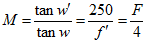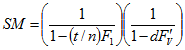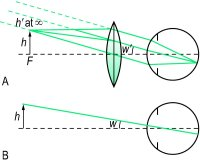# magnification

(redirected from Linear magnification)
Also found in: Dictionary, Thesaurus, Encyclopedia.
Related to Linear magnification: Angular magnification

## magnification

[mag″nĭ-fĭ-ka´shun]
1. apparent increase in size, as under the microscope.
2. the process of making something appear larger, as by use of lenses.
3. the ratio of apparent (image) size to real size.
Miller-Keane Encyclopedia and Dictionary of Medicine, Nursing, and Allied Health, Seventh Edition. © 2003 by Saunders, an imprint of Elsevier, Inc. All rights reserved.

## mag·ni·fi·ca·tion

(mag'ni-fi-kā'shŭn),
1. The seeming increase in size of an object viewed under the microscope; when noted, this increased size is expressed by a figure preceded by ×, indicating the number of times its diameter is enlarged.
2. The increased amplitude of a tracing, as of a muscular contraction, caused by the use of a lever with a long writing arm, that is, one in which the fulcrum is placed nearer to the muscle than to the writing point.
[L. magnifico, pp. -atus, to magnify]
Farlex Partner Medical Dictionary © Farlex 2012

## magnification

Amplification of an image.

## magnification

Imaging Amplification of an image. See Biologic magnification, Microscopy.
McGraw-Hill Concise Dictionary of Modern Medicine. © 2002 by The McGraw-Hill Companies, Inc.

## mag·ni·fi·ca·tion

(mag'ni-fi-kā'shŭn)
1. The seeming increase in size of an object viewed under the microscope; when written, this increased size is expressed by a figure preceded by ×, indicating the number of times its diameter is enlarged.
2. The increased amplitude of a tracing, as of a muscular contraction, caused by the use of a lever with a long writing arm.
[L. magnifico, pp. -atus, to magnify]
Medical Dictionary for the Health Professions and Nursing © Farlex 2012

## magnification

1. An increase in the apparent size of an object. Syn. enlargement. 2. Specification of a magnifying device to form an enlarged image.
angular magnification Magnification expressed as the ratio of the angle α′ subtended at the eye by the image to the angle α subtended at the eye by the object (assuming small angles)
M = tan α′/tan α
Syn. angular enlargement. Note: low vision practitioners consider this type of magnification in which no specific distance is specified as a synonym of apparent magnification.
apparent magnification Magnification produced by a viewing instrument or lens expressed as the ratio of the angle w′ subtended at the nodal point of the eye by the image, to the angle w subtended at the nodal point by the object, when placed at a standard (reference) distance called 'the least distance of distinct vision' from the unaided eye. It is conventional to take this distance as 250 mm and to place the object in the anterior focal plane of the magnifying device. The magnification M is, then, equal to (assuming small angles)where f′ and F are the second focal length (in mm) and power (in dioptres) of the magnifying device, respectively (Fig. M4). In this object location the magnification (and therefore the retinal image size) is constant and independent of the distance between the magnifier and the eye, but the field of view decreases as the distance between the eye and the magnifier increases. Syn. conventional magnification; effective magnification; loupe magnification; nominal magnification; relative magnification; standard magnification.If the object is closer to the magnifying device than its anterior focal plane so that its image is formed at the least distance of distinct vision (25 cm), and assuming that the eye is so close to the magnifier as to ignore the distance separating them and that the patient has an accommodation (or a near addition) of +4.00 D, the magnification M is, then, equal to
M = 1 + (F/4)
Example: a lens of +16.00 D provides, in these conditions, a magnification of 5✕. Syn. iso-accommodative magnification; magnifying power; trade magnification. See iso-accommodative magnification; lateral magnification; equivalent viewing power.
axial magnification The ratio of the distance along the optical axis between two points in image space l′ to the distance along the optical axis between the corresponding two points in object space l, i.e. l′/l. The axial magnification is approximately equal to the square of the lateral magnification when the object is far away from the optical system. This magnification is useful when considering an image in its three dimensions. Clinically, it is important when assessing the thickness of a retinal lesion in indirect ophthalmoscopy. Syn. longitudinal magnification.
combined magnification The product of the individual values of each type of magnification used in combination with each other. Example: if a patient uses a CCTV monitor to provide a magnification of 5✕ viewed at a distance of 50 cm, and then views the same screen at a distance of 25 cm, thus producing a relative distance magnification of 50/25 = 2✕, the total magnification is 5 ✕ 2 = 10✕. Syn. total magnification.
conventional magnification See apparent magnification.
cortical magnification Term referring to the fact that the amount of cortical area devoted to processing visual information from the central area of the retina far exceeds the amount devoted to the peripheral retina. It is estimated that about 25% of the cells in the visual cortex are devoted to processing the central 2.5º of the visual field. Syn. magnification factor. See visual area; macular sparing.
distance magnification See relative distance magnification.
effective magnification See apparent magnification.
electronic magnification Magnification obtained using an electronic vision enhancement system (EVES), such as a closed-circuit television (CCTV). It is equal to the ratio of the size of the image on the screen to the size of the original object being viewed. Example: an object 2cm in height measures 6 cm on the screen, the magnification is 6/2 = 3✕. Syn. real image magnification; transverse magnification.
magnification factor See cortical magnification.
iso-accommodative magnification The magnification of a lens (or lens system) when the distance of the image from the eye (or spectacle plane) formed by a magnifier is equal to the distance of the object from the eye viewed without the magnifier. Thus the same amount of accommodation (or near addition) is required with or without the magnifier. It is equal to
M = 1 + (F/D)
where F is the power of the magnifier (assumed to be so close to the eye as to ignore the distance separating them) and D the object vergence. The special case in which the object distance from the eye is 25 cm (D = 4.00 D) is the trade magnification. See apparent magnification.
lateral magnification Magnification of a lens or of an optical system, expressed as the ratio of the size of the image h′ to the size of the object h. It is usually denoted by
M = h′/h = l′/l = L/L
where l′ and l are the distances of the image and object, respectively from the principal plane of the lens (or lens system) and L and L′ the object and image vergences, respectively. Syn. enlargement ratio (ER); linear magnification; transverse magnification. (Note: some authors consider this last term a synonym of electronic magnification.) See equivalent viewing power.
linear magnification See lateral magnification.
longitudinal magnification See axial magnification.
loupe magnification See apparent magnification.
negative magnification See minification.
nominal magnification See apparent magnification.
magnification power See spectacle magnification.
real image magnification See electronic magnification.
relative magnification See apparent magnification.
relative distance magnification The magnification that results from decreasing the distance between an object and the eye. It is expressed as
Md
= x/x′where x and x′ are the initial distance and the new distance, respectively. Example: if the viewing distance is decreased from 60 cm to 20 cm, Md = 60/20 = 3✕. Syn. distance magnification; relative distance enlargement.
relative size magnification The magnification which results from increasing the actual size of an object viewed. Examples: a larger TV screen; a larger print book than one used previously. It is expressed as
Ms
= h2/h1where h2 and h1 are the sizes of the enlarged object and the initial object, respectively. Syn. size magnification; relative size enlargement.
relative spectacle magnification (RSM) The ratio of the retinal image size in the corrected ametropic eye to that in a standard emmetropic eye. See Knapp's law.
shape magnification Magnification resulting from a variation in the curvature of the front surface and thickness of an ophthalmic lens. In the treatment of aniseikonia it may be necessary to alter the magnification of a lens while leaving its dioptric power unchanged. Syn. shape factor. See aniseikonic lens; spectacle magnification.
size magnification See relative size magnification.
spectacle magnification The ratio of the retinal image of a distant object in the corrected ametropic eye to the blurred or sharp image formed in the same eye when uncorrected. It is greater than unity in the hyperopic eye, and less than unity in myopia. With a contact lens, though, this magnification is nearly equal to unity whatever the refractive error. Spectacle magnification SM depends both on the shape of the spectacle lens (i.e. the power of its front surface and its thickness) and on the power of the lens. Thuswhere F1 is the power of the front surface, Fv the back vertex power of the lens, t its thickness, n the index of refraction and d the distance from the back surface of the lens to the entrance pupil of the eye. The first term in the formula represents the shape factor (shape magnification) and the second term the power factor (power magnification). However, since the shape factor is very small for most common ophthalmic lenses (except for high plus lenses), it is often ignored in the above formula.
telescopic magnification Magnification obtained with a telescope, such as a galilean telescope which gives an erect image or an astronomical telescope in which an erecting system is used. The magnification is
M = α′/α = −Fe /Fo
where α′ and α are the angles subtended at the eye by the image viewed through the telescope and the angle subtended at the eye by the object, respectively and Fe and Fo are the powers of the eyepiece and objective, respectively. Telescopes are used to magnify objects at distance (afocal) and placed over the spectacle correction. If the patient is uncorrected the telescope can be adjusted but the magnification will change. They can be used for near and intermediate viewing by altering the distance between the objective and the eyepiece, or adding a plus lens in front of the objective, the result being a combined magnification (afocal telescope magnification ✕ power of the plus lens ÷ 4).
total magnification See combined magnification.
transverse magnification See electronic magnification; linear magnification.Fig. M4 Object h viewed, A, with a loupe and placed at its anterior principal focus F, and B, without

 Table M1 Apparent magnification (or conventional magnification) of microscopic lenses of various powers used in the correction of low vision and their corresponding reading distance (assuming emmetropia or correction for distance and that no accommodation is exerted) magnification lens power (D) reading distance (cm) 1✕ +4.00 25 1.5✕ +6.00 16.7 2✕ +8.00 12.5 2.5✕ +10.00 10 3✕ +12.00 8.3 4✕ +16.00 6.25 5✕ +20.00 5 6✕ +24.00 4.2 8✕ +32.00 3.12 10✕ +40.00 2.5

 Table M2 Approximate lateral magnification (in %) corresponding to various changes in spectacle lens distance and for various lens powers distance change lens power (D) 1 2 4 6 8 10 1 mm 0.1 0.2 0.4 0.6 0.8 1.0 2 mm 0.2 0.4 0.8 1.2 1.6 2.0 3 mm 0.3 0.6 1.2 1.8 2.4 3.0 4 mm 0.4 0.8 1.6 2.4 3.2 4.0 5 mm 0.5 1.0 2.0 3.0 4.0 5.0 10 mm 1.0 2.0 4.0 6.0 8.0 10.0 minus lens: if moved closer to the eye magnification increases; if moved further from the eye, magnification decreases plus lens: if moved closer to the eye magnification decreases; if moved further from the eye, magnification increases

 Table M3 Approximate spectacle magnification of lenses of various back vertex powers (F′v in D) assuming d = 15 mm and the parameters of a typical spectacle lens of that power F′v shape factor (S) power factor (P) spectacle magnification (SM) percentage magnification +12 1.08 1.22 1.32 32% increase +10 1.06 1.18 1.25 25% ” +8 1.04 1.14 1.18 18% ” +6 1.03 1.10 1.13 13% ” +4 1.02 1.06 1.09 9% ” +2 1.01 1.03 1.04 4% ” 0 1.01 1.00 1.01 1% ” −2 1.00 0.97 0.97 3% decrease −4 1.00 0.94 0.94 6% ” −6 1.00 0.92 0.92 8% ” −8 1.00 0.89 0.89 11% ” −10 1.00 0.87 0.87 13% ” −12 1.00 0.85 0.85 15% ” −14 1.00 0.83 0.83 17% ” −16 1.00 0.81 0.81 19% ”

 Table M4 Approximate spectacle and contact lens magnification assuming d = 15 mm (vertex distance 12 mm plus 3 mm between cornea and entrance pupil) and negligible lens thickness. The percentage change in magnification going from spectacles to contact lenses was calculated using (Fs/Fc − 1) ✕ 100 spectacle refraction (Fs) equivalent power of contact lens (Fc) percentage increased magnification spectacles percentage increased magnification contact lens % change in magnification from specs. to contact lens +12 +14.02 21.9 4.4 −14.4 +10 +11.36 17.6 3.5 −12 +8 +8.85 13.6 2.7 −9.6 +6 +6.47 9.9 2.0 −7.3 +4 +4.2 6.4 1.3 −4.8 +2 +2.05 3.1 0.6 −2.4 −2 −1.95 −2.9 −0.6 2.6 −4 −3.82 −5.7 −1.1 4.7 −6 −5.6 −8.3 −1.7 7.1 −8 −7.3 −10.7 −2.1 9.6 −10 −8.93 −13 −2.6 12 −12 −10.49 −15.3 −3 14.4 −14 −11.99 −17.3 −3.5 16.8 −16 −13.42 −19.3 −3.9 19.2
Millodot: Dictionary of Optometry and Visual Science, 7th edition. © 2009 Butterworth-Heinemann
Site: Follow: Share:
Open / Close